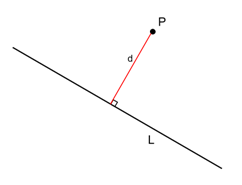# Image of the point P with position vector,has the position vector: Option 1) (-9,5,2) Option 2) (9,5,-2) Option 3) (9,-5,-2) Option 4) None

As learnt in

Perpendicular distance of a point from a line, foot of perpendicular and image (Cartesian form ) - - whereinL be the foot of perpendicular drawn fromon the lineCo-ordinates of L will beDR's of PL will bePL is perpendicular to line,Findand put in coordinates of L Distance between P and L is perpendicular distance Image Q can be obtained by applying mid point formula in P and Q and equating it to L obtained.We have equation of the line in cartesian form:lie on the given line.

So,So,Putting iv (i),So,Option 1)

(-9,5,2)

This solution is incorrect

Option 2)

(9,5,-2)

This solution is correct

Option 3)

(9,-5,-2)

This solution is incorrect

Option 4)

None

This solution is incorrect

## Most Viewed Questions

### Preparation Products

##### Knockout BITSAT 2021

It is an exhaustive preparation module made exclusively for cracking BITSAT..

₹ 4999/- ₹ 2999/-